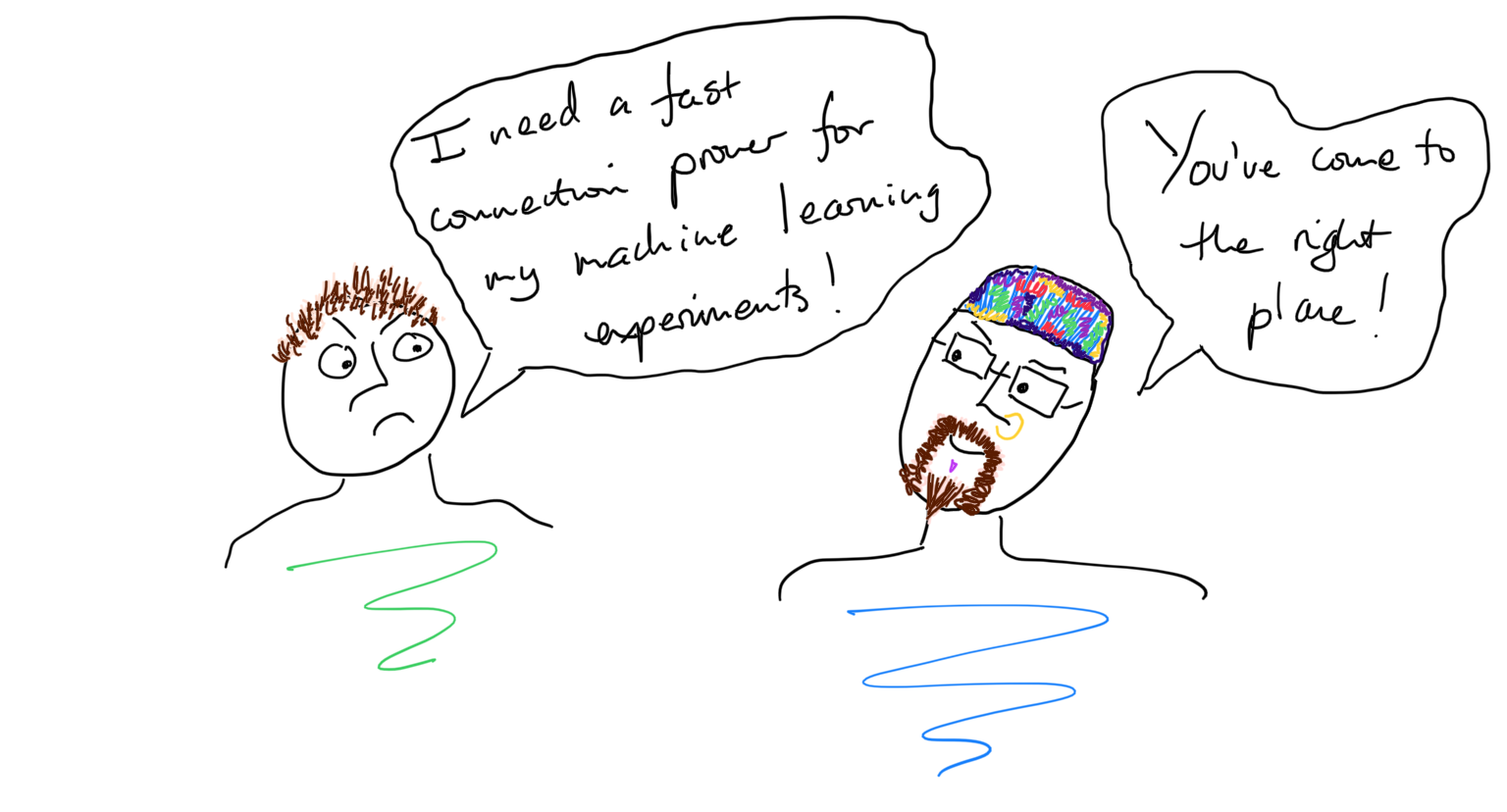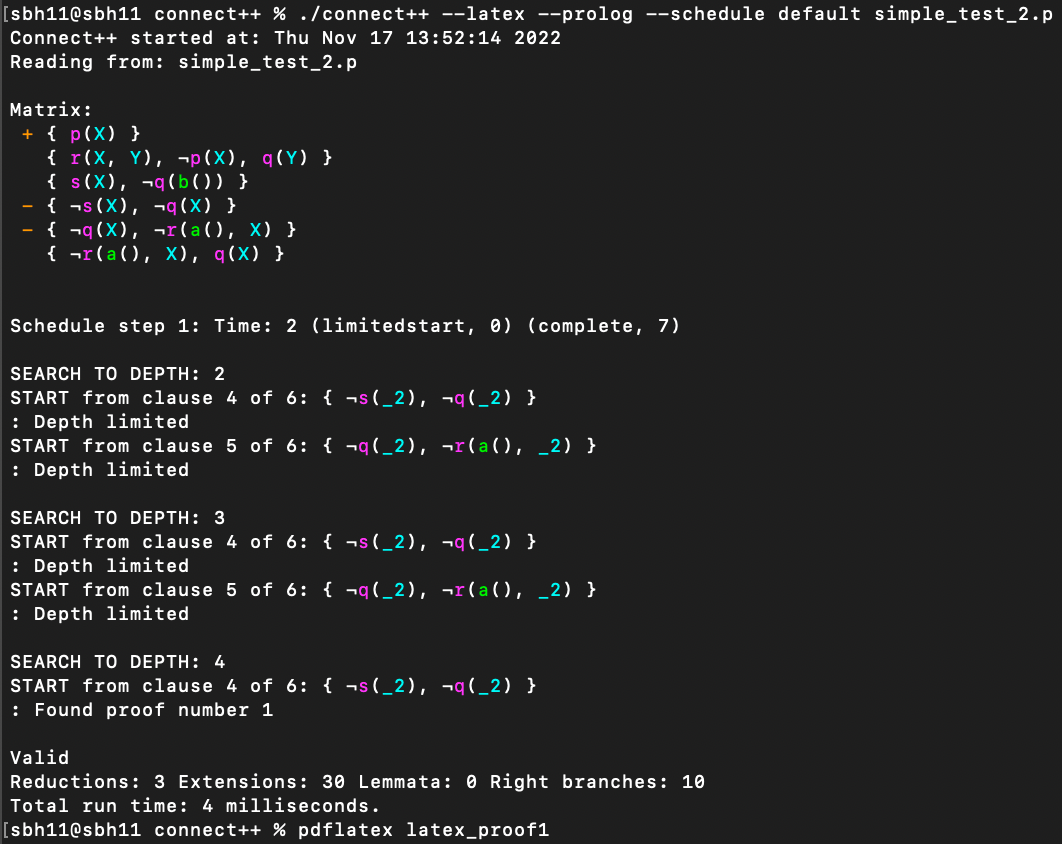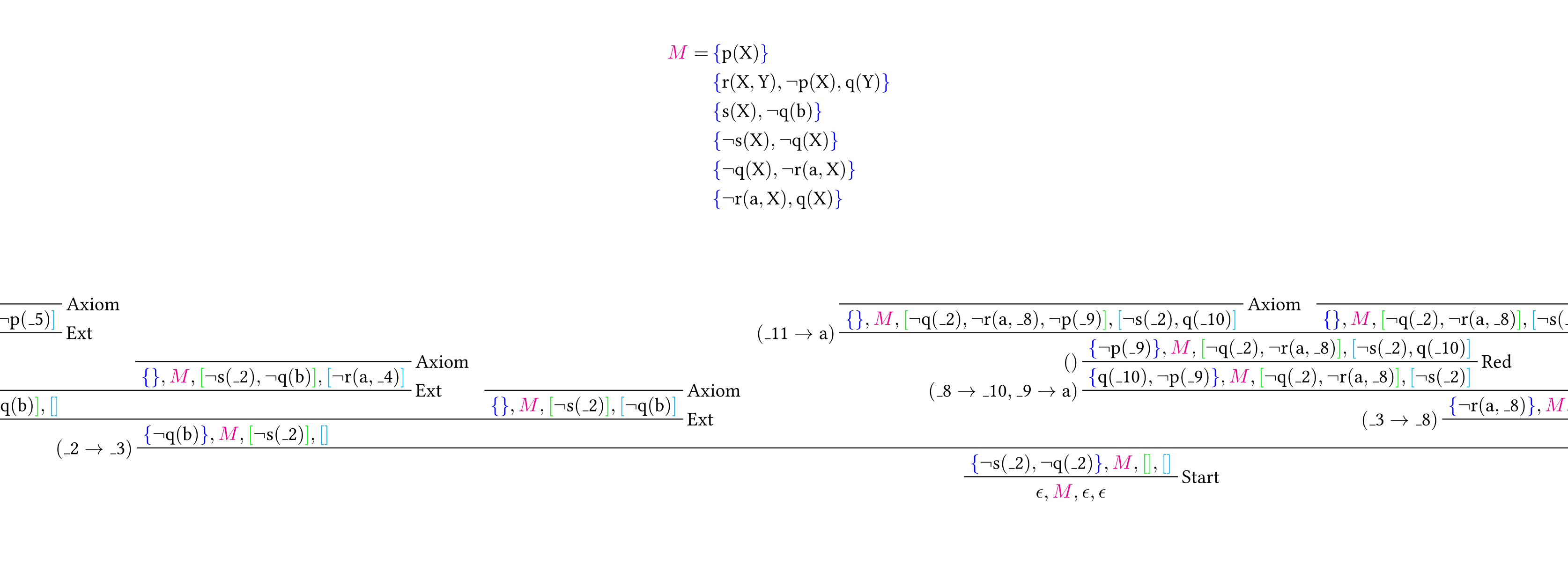Dr Sean Holden
University Associate Professor
of Computer Science

# Email

sbh11 at cl.cam.ac.uk

Please use the @cl form of address in preference to any other you may have. (Executive summary: the University decided to farm out its email provision to Micro\$oft, with disasterous consequences. By using the correct email address your message should be handled by a proper, standards-compliant alternative, and therefore stands a much better chance of reaching me.)

University of Cambridge
Department of Computer Science and Technology
The Computer Laboratory
William Gates Building
15 JJ Thomson Avenue
Cambridge
CB3 0FD

Trinity College
Cambridge
CB2 1TQ

# Phone

+44 (0)1223 763725 (University)
+44 (0)1223 338452 (College)

# Orcid

0000-0001-7979-1148

# Also available...

...on DBLP and Semantic Scholar.

If you want to study with me, please read the FAQs before doing anything else.

# Teaser...Lean theorem provers are great: who doesn't want something implemented in a few lines of Prolog? However, they have some definite problems:

• The automated theorem-proving (ATP) world likes to see things going faster and/or more things being proved. If your prover is implemented in Prolog it starts at a disadvantage.
• Many of the best heuristics involve getting control over the backtracking process, and this is not necessarily straightforward if you want something better than cut.
• There are lots of other ways you might incorporate good heuristics into the proof search, but influencing a Prolog-based search in the way you want to may not be straightforward.
• If you want to use machine learning, then getting Prolog, Python and C++ to play nicely together on a high-performance computing facility can be, for want of a better word, frustrating.

Connect++ is a connection prover implemented in C++ specifically with the aim of making it fast, and easy to incorporate new heuristics and machine learning methods.

Connect++ is currently a work in progress but my aim is to open-source it in 2023. Here's a bit of a teaser...

## Step 1: present your problem in TPTP format

% % Problem from "Guiding Inference in Connection Tableaux % by Recurrent Neural Networks", Piotrowski and Urban, % CICM 2020. % cnf(c1, unknown, p(X)). cnf(c2, unknown, r(X,Y) | ~p(X) | q(Y) ). cnf(c3, unknown, s(X) | ~q(b) ). cnf(c4, unknown, ~s(X) | ~q(X) ). cnf(c5, unknown, ~q(X) | ~r(a,X) ). cnf(c6, unknown, ~r(a,X) | q(X) ).

You can find the specification for the TPTP format here.

## Step 2: define a schedule

You can run Connect++ using a large number of options for parameters related to start clause selection, restriction of backtracking and so on.

The use of --schedule default on the command line directs Connect++ to use a straightforward schedule rather than fixed parameters. At present the default schedule looks like this:

sbh11@sbh11 resources % more default_schedule.txt 2 limitedstart complete 7 ; 60 conjecturestart nocomplete ; 20 limitedstart restrictstart nocomplete ; 2 conjecturestart reorder 23 nocomplete ; 2 limitedstart restrictstart reorder 29 nocomplete ; 2 conjecturestart reorder 37 nocomplete ; 2 limitedstart restrictstart reorder 41 nocomplete ; 2 conjecturestart reorder 47 nocomplete ; 0 limitedstart allbacktrack nocomplete ; sbh11@sbh11 resources %

But you can specify a file containing your own schedule and Connect++ will parse it.

## Step 3: run the prover

Unsurprisingly, it doesn't have too much trouble with the example quoted above:## Step 4: take a look at the proofThe use of --latex on the command line directs Connect++ to produce a LaTeX representation of the first proof it finds.

Proofs tend to be wide, so this illustration is cropped. The full diagram is here.

## Step 5: verify the proof's certificate

The use of --prolog on the command line directs Connect++ to produce a certificate for the first proof it finds.

This can be checked by a short Prolog program:

Start Clause: [-s(_13892),-q(_13892)] ---------- Left extension: depth = 2 C2 = [s(_13976),-q(b)] L2 = s(_13976) Unification OK. C = [-q(b)] P = [-s(_13892)] L = [] ---------- Left extension: depth = 3 C2 = [-r(a,_18196),q(_18196)] L2 = q(_18196) Unification OK. C = [-r(a,b)] P = [-q(b),-s(_13892)] L = [] ---------- Left extension: depth = 4 C2 = [r(_18406,_18408),-p(_18406),q(_18408)] L2 = r(_18406,_18408) Unification OK. C = [q(b),-p(a)] P = [-r(a,b),-q(b),-s(_13892)] L = [] ---------- Reduction: Lit resolved in path: -q(b) Unification OK. C = [-p(a)] P = [-r(a,b),-q(b),-s(_13892)] L = [q(b)] ---------- Left extension: depth = 6 C2 = [p(_19182)] L2 = p(_19182) Unification OK. C = [] P = [-p(a),-r(a,b),-q(b),-s(_13892)] L = [q(b)] ---------- Axiom ---------- Right extension: depth = 6 C = [] P = [-r(a,b),-q(b),-s(_13892)] L = [-p(a),q(b)] ---------- Axiom ---------- Right extension: depth = 4 C = [] P = [-q(b),-s(_13892)] L = [-r(a,b)] ---------- Axiom ---------- Right extension: depth = 3 C = [] P = [-s(_13892)] L = [-q(b)] ---------- Axiom ---------- Right extension: depth = 2 C = [-q(_13892)] P = [] L = [-s(_13892)] ---------- Left extension: depth = 3 C2 = [-r(a,_19704),q(_19704)] L2 = q(_19704) Unification OK. C = [-r(a,_13892)] P = [-q(_13892)] L = [-s(_13892)] ---------- Left extension: depth = 4 C2 = [r(_19914,_19916),-p(_19914),q(_19916)] L2 = r(_19914,_19916) Unification OK. C = [q(_13892),-p(a)] P = [-r(a,_13892),-q(_13892)] L = [-s(_13892)] ---------- Reduction: Lit resolved in path: -q(_13892) Unification OK. C = [-p(a)] P = [-r(a,_13892),-q(_13892)] L = [q(_13892),-s(_13892)] ---------- Left extension: depth = 6 C2 = [p(_20232)] L2 = p(_20232) Unification OK. C = [] P = [-p(a),-r(a,_13892),-q(_13892)] L = [q(_13892),-s(_13892)] ---------- Axiom ---------- Right extension: depth = 6 C = [] P = [-r(a,_13892),-q(_13892)] L = [-p(a),q(_13892),-s(_13892)] ---------- Axiom ---------- Right extension: depth = 4 C = [] P = [-q(_13892)] L = [-r(a,_13892),-s(_13892)] ---------- Axiom ---------- Right extension: depth = 3 C = [] P = [] L = [-q(_13892),-s(_13892)] ---------- Axiom ---------- true .

## Step 6: improve the process by adding some machine learning

This is the reason I wrote Connect++ in the first place! There is of course existing literature addressing this, but there's also plenty of room for new things.

## Step 7: profit...

Still working on this part...